# Running multiple HPO jobs in parallel on Amazon SageMaker

The ability to rapidly iterate and train machine learning (ML) models is key to deriving business value from ML workloads. Because ML models often have many tunable parameters (known as hyperparameters) that can influence the model’s ability to effectively learn, data scientists often use a technique known as hyperparameter optimization (HPO) to achieve the best-performing model against a certain predefined metric. Depending on the number of hyperparameters and the size of the search space, finding the best model can require thousands or even tens of thousands of training runs. Real-world problems that often require extensive HPO include image segmentation for modeling vehicular traffic for autonomous driving, developing algorithmic trading strategies based on historical financial data, or building fraud detection models on transaction data. Amazon SageMaker provides a built-in HPO algorithm that removes the undifferentiated heavy lifting required to build your own HPO algorithm. This post shows how to batch your HPO jobs to maximize the number of jobs you can run in parallel, thereby reducing the total time it takes to effectively cover the desired parameter space and obtain the best-performing models.

Before diving into the batching approach on Amazon SageMaker, let’s briefly review the state-of-the-art . There are a large number of HPO algorithms, ranging from random or grid search, Bayesian search, and hand tuning, where researchers use their domain knowledge to tune parameters to population-based training inspired from genetic algorithms. For deep learning models, however, even training a single training run can be time consuming. In that case, it becomes important to have an aggressive early stopping strategy, which ends trials in search spaces that are unlikely to produce good results. Several strategies like successive halving or asynchronous successive halving use multi-arm bandits to trade-off between exploration (trying out different parameter combinations) versus exploitation (allowing a training run to converge). Finally, to help developers quickly iterate with these approaches, there are a number of tools, such as SageMaker HPO, Ray, HyperOpt, and more. In this post, you also see how you can bring one of the most popular HPO tools, Ray Tune, to SageMaker.

## Use case: Predicting credit card loan defaults

To demonstrate this on a concrete example, imagine that you’re an ML engineer working for a bank, and you want to predict the likelihood of a customer defaulting on their credit card payments. To train a model, you use historical data available from the UCI repository. All the code developed in this post is made available on GitHub. The notebook covers the data preprocessing required to prep the raw data for training. Because the number of defaults is quite small (as shown in the following graph), we split the dataset into train and test, and upsample the training data to 50/50 default versus non-defaulted loans.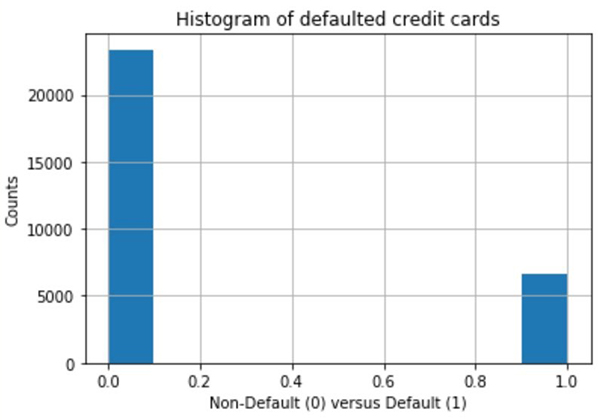Then we upload the datasets to Amazon Simple Storage Service (Amazon S3). See the following code:

``````#Upload Training and test data into S3
train_s3 = sagemaker_session.upload_data(path='./train_full_split/', key_prefix=prefix + '/train')
print(train_s3)
test_s3 = sagemaker_session.upload_data(path='./test_full_split/', key_prefix=prefix + '/test')
print(test_s3)``````

Although SageMaker provides many built-in algorithms, such as XGBoost, in this post we demonstrate how to apply HPO to a custom PyTorch model using the SageMaker PyTorch training container using script mode. You can then adapt this to your own custom deep learning code. Furthermore, we will demonstrate how you can bring custom metrics to SageMaker HPO.

When dealing with tabular data, it’s helpful to shard your dataset into smaller files to avoid long data loading times, which can starve your compute resources and lead to inefficient CPU/GPU usage. We create a custom Dataset class to fetch our data and wrap this in the DataLoader class to iterate over the dataset. We set the batch size to 1, because each batch consists of 10,000 rows, and load it using Pandas.

Our model is a simple feed-forward neural net, as shown in the following code snippet:

``````class Net(nn.Module):
def __init__(self, inp_dimension):
super().__init__()
self.fc1 = nn.Linear(inp_dimension, 500)
self.drop = nn.Dropout(0.3)
self.bn1 = nn.BatchNorm1d(500)
self.bn2=nn.BatchNorm1d(250)
self.fc2 = nn.Linear(500, 250)
self.fc3 = nn.Linear(250, 100)
self.bn3=nn.BatchNorm1d(100)
self.fc4 = nn.Linear(100,2)

def forward(self, x):
x = x.squeeze()
x = F.relu(self.fc1(x.float()))
x = self.drop(x)
x = self.bn1(x)
x = F.relu(self.fc2(x))
x = self.drop(x)
x = self.bn2(x)
x = F.relu(self.fc3(x))
x = self.drop(x)
x = self.bn3(x)
x = self.fc4(x)
# last layer converts it to binary classification probability
return F.log_softmax(x, dim=1)``````

As shown in the Figure above, the dataset is highly imbalanced and as such, model accuracy isn’t the most useful evaluation metric, because a baseline model that predicts all customers won’t default on their payments will have high accuracy. A more useful metric is the AUC, which is the area under the receiver operator characteristic (ROC) curve that aims to minimize the number of false positives while maximizing the number of true positives. A false positive (model incorrectly predicting a good customer will default on their payment) can cause the bank to lose revenue by denying credit cards to customers. To make sure that your HPO algorithm can optimize on a custom metric such as the AUC or F1-score, you need to log those metrics into STDOUT, as shown in the following code:

``````def test(model, test_loader, device, loss_optim):
model.eval()
test_loss = 0
correct = 0
fulloutputs = []
fulltargets = []
fullpreds = []
for i, (data, target) in enumerate(test_loader):
data, target = data.to(device), target.to(device)
output = model(data)
target = target.squeeze()
test_loss += loss_optim(output, target).item()  # sum up batch loss
pred = output.max(1, keepdim=True)  # get the index of the max log-probability
correct += pred.eq(target.view_as(pred)).sum().item()
fulloutputs.append(output.cpu().numpy()[:, 1])
fulltargets.append(target.cpu().numpy())
fullpreds.append(pred.squeeze().cpu())

i+=1
test_loss /= i
logger.info("Test set Average loss: {:.4f}, Test Accuracy: {:.0f}%;n".format(
test_loss, 100. * correct / (len(target)*i)
))
fulloutputs = [item for sublist in fulloutputs for item in sublist]
fulltargets = [item for sublist in fulltargets for item in sublist]
fullpreds = [item for sublist in fullpreds for item in sublist]
logger.info('Test set F1-score: {:.4f}, Test set AUC: {:.4f} n'.format(
f1_score(fulltargets, fullpreds), roc_auc_score(fulltargets, fulloutputs)))``````

Now we’re ready to define our SageMaker estimator and define the parameters for the HPO job:

``````estimator = PyTorch(entry_point="train_cpu.py",
role=role,
framework_version='1.6.0',
py_version='py36',
source_dir='./code',
output_path = f's3://{bucket}/{prefix}/output',
instance_count=1,
sagemaker_session=sagemaker_session,
instance_type='ml.m5.xlarge',
hyperparameters={
'epochs': 10, # run more epochs for HPO.
'backend': 'gloo' #gloo for cpu, nccl for gpu
}
)

#specify the hyper-parameter ranges
hyperparameter_ranges = {'lr': ContinuousParameter(0.001, 0.1),
'momentum': CategoricalParameter(list(np.arange(0, 10)/10))}

inputs ={'training': train_s3,
'testing':test_s3}

#specify the custom HPO metric
objective_metric_name = 'test AUC'
objective_type = 'Maximize'
metric_definitions = [{'Name': 'test AUC',
'Regex': 'Test set AUC: ([0-9\.]+)'}]   #note that the regex must match your test function above
estimator.fit({'training': train_s3,
'testing':test_s3},
wait=False)
``````

We pass in the paths to the training and test data in Amazon S3.

With the setup in place, let’s now turn to running multiple HPO jobs.

## Parallelizing HPO jobs

To run multiple hyperparameter tuning jobs in parallel, we must first determine the tuning strategy. SageMaker currently provides a random and Bayesian optimization strategy. For random strategy, different HPO jobs are completely independent of one another, whereas Bayesian optimization treats the HPO problem as a regression problem and makes intelligent guesses about the next set of parameters to pick based on the prior set of trials.

First, let’s review some terminology:

• Trials – A trial corresponds to a single training job with a set of fixed values for the hyperparameters
• max_jobs – The total number of training trials to run for that given HPO job
• max_parallel_jobs – The maximum concurrent running trials per HPO job

Suppose you want to run 10,000 total trials. To minimize the total HPO time, you want to run as many trials as possible in parallel. This is limited by the availability of a particular Amazon Elastic Compute Cloud (Amazon EC2) instance type in your Region and account. If you want to modify or increase those limits, speak to your AWS account representatives.

For this example, let’s suppose that you have 20 ml.m5.xlarge instances available. This means that you can simultaneously run 20 trials of one instance each. Currently, without increasing any limits, SageMaker limits `max_jobs` to 500 and `max_parallel_jobs` to 10. This means that you need to run a total of 10,000/500 = 20 HPO jobs. Because you can run 20 trials and max_parallel_jobs is 10, you can maximize the number of simultaneous HPO jobs running by running 20/10 = 2 HPO jobs in parallel. So one approach to batch your code is to always have two jobs running, until you meet your total required jobs of 20.

In the following code snippet, we show two ways in which you can poll the number of running jobs to achieve this. The first approach uses boto3, which is the AWS SDK for Python to poll running HPO jobs, and can be run in your notebook and is illustrated pictorially in the following diagram. This approach can primarily be used by data scientists. Whenever the number of running HPO jobs falls below a fixed number, indicated by the blue arrows in the dashed box on the left, the polling code will launch new jobs (shown in orange arrows). The second approach uses Amazon Simple Queue Service (Amazon SQS) and AWS Lambda to queue and poll SageMaker HPO jobs, allowing you to build an operational pipeline for repeatability.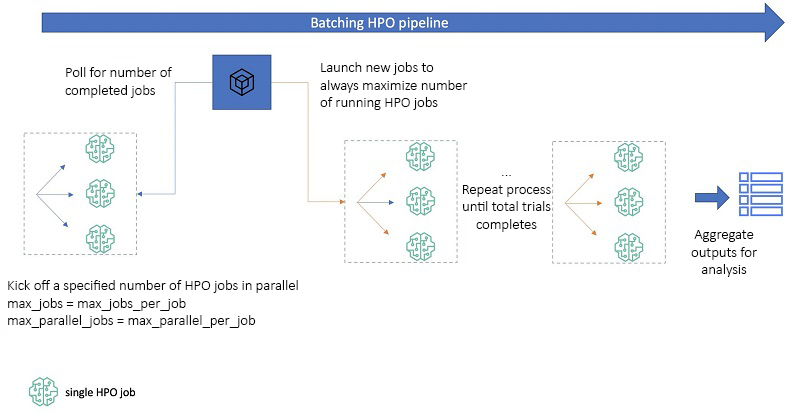Sounds complicated? No problem, the following code snippet allows you to determine the optimal strategy to minimize your overall HPO time by running as many HPO jobs in parallel as allowed. After you determine the instance type you want to use and your respective account limits for that instance, replace `max_parallel_across_jobs` with your value.

``````def bayesian_batching_cold_start(total_requested_trials, max_parallel_across_jobs=20, max_parallel_per_job=10, max_candidates_per_job = 500):
'''Given a total number of requested trials, generates the strategy for Bayesian HPO
The strategy is a list (batch_strat) where every element is the number of jobs to run in parallel. The sum of all elements in the list is
the total number of HPO jobs needed to reach total_requested_trials. For example if batch_strat = [2, 2, 2, 1], means you will run a total of 7
HPO jobs starting with 2 --> 2 ---> 2 ---> 1.
total_requested_trials = number of trails user wants to run.
max_parallel_across_jobs = max number of training jobs across all trials Sagemaker runs in parallel. Limited by instance availability
max_parallel_per_job = max number of parallel jobs to run per HPO job
max_candidates_per_job = total number of training jobs per HPO job'''
batch_strat = []
tot_jobs_left = total_requested_trials
max_parallel_hpo_jobs = max_parallel_across_jobs//max_parallel_per_job
if total_requested_trials < max_parallel_hpo_jobs*max_candidates_per_job:
batch_strat.append(total_requested_trials//max_candidates_per_job)
else:
while tot_jobs_left > max_parallel_hpo_jobs*max_candidates_per_job:
batch_strat.append(max_parallel_hpo_jobs)
tot_jobs_left -=max_parallel_hpo_jobs*max_candidates_per_job

batch_strat.append(math.ceil((tot_jobs_left)/max_candidates_per_job))
return math.ceil(total_requested_trials/max_candidates_per_job), max_parallel_hpo_jobs, batch_strat

bayesian_batching_cold_start(10000)
(20, 2, [2, 2, 2, 2, 2, 2, 2, 2, 2, 2])
``````

After you determine how to run your jobs, consider the following code for launching a given sequence of jobs. The helper function `_parallel_hpo_no_polling` runs the group of parallel HPO jobs indicated by the dashed box in the preceding figure. It’s important to set the `wait` parameter to `False` when calling the tuner, because this releases the API call to allow the loop to run. The orchestration code `poll_and_run` polls for the number of jobs that are running at any given time. If the number of jobs falls below the user-specified maximum number of trials they want to run in parallel (`max_parallel_across_jobs`), the function automatically launches new jobs. Now you might be thinking,  “But these jobs can take days to run, what if I want to turn off my laptop or if I lose my session?” No problem, the code picks up where it left off and runs the remaining number of jobs by counting how many HPO jobs are remaining prefixed by the job_name_prefix you provide.

Finally, the `get_best_job` function aggregates the outputs in a Pandas DataFrame in ascending order of the objective metric for visualization.

``````# helper function to launch a desired number of "n_parallel" HPO jobs simultaneously
def _parallel_hpo_no_polling(job_name_prefix, n_parallel, inputs, max_candidates_per_job, max_parallel_per_job):
"""kicks off N_parallel Bayesian HPO jobs in parallel
job_name_prefix: user specified prefix for job names
n_parallel: Number of HPO jobs to start in parallel
inputs: training and test data s3 paths
max_candidates_per_job: number of training jobs to run in each HPO job in total
max_parallel_per_job: number of training jobs to run in parallel in each job

"""
# kick off n_parallel jobs simultaneously and returns all the job names
tuning_job_names = []
for i in range(n_parallel):
timestamp_suffix = strftime("%d-%H-%M-%S", gmtime())
try:
tuner = HyperparameterTuner(estimator,
objective_metric_name,
hyperparameter_ranges,
metric_definitions,
max_jobs=max_candidates_per_job,
max_parallel_jobs=max_parallel_per_job,
objective_type=objective_type
)
# fit the tuner to the inputs and include it as part of experiments
tuner.fit(inputs,
job_name = f'{job_name_prefix}-{timestamp_suffix}',
wait=False
) # set wait=False, so you can launch other jobs in parallel.
tuning_job_names.append(tuner.latest_tuning_job.name)
sleep(1) #this is required otherwise you will get an error for using the same tuning job name
print(tuning_job_names)
except Exception as e:
sleep(5)
return tuning_job_names

#orchestration and polling logicdef poll_and_run(job_name_prefix, inputs, max_total_candidates, max_parallel_across_jobs, max_candidates_per_job, max_parallel_per_job):
"""Polls for number of running HPO jobs. If less than max_parallel , starts a new one.
job_name_prefix: the name prefix to give all your training jobs
max_total_candidates: how many total trails to run across all HPO jobs
max_candidates_per_job: how many total trails to run for 1 HPO job
max_parallel_per_job: how many trials to run in parallel for a given HPO job (fixed to 10 without limit increases).
max_parallel_across_jobs: how many concurrent trials to run in parallel across all HPO jobs
"""
#get how many jobs to run in total and concurrently
max_num, max_parallel, _ = bayesian_batching_cold_start(max_total_candidates,
max_parallel_across_jobs=max_parallel_across_jobs,
max_parallel_per_job=max_parallel_per_job,
max_candidates_per_job = max_candidates_per_job
)

# continuously polls for running jobs -- if they are less than the required number, then launches a new one.

all_jobs = sm.list_hyper_parameter_tuning_jobs(SortBy='CreationTime', SortOrder='Descending',
NameContains=job_name_prefix,
MaxResults = 100)['HyperParameterTuningJobSummaries']
all_jobs = [i['HyperParameterTuningJobName'] for i in all_jobs]

if len(all_jobs)==0:
print(f"Starting a set of HPO jobs with the prefix {job_name_prefix} ...")
num_left = max_num
#kick off the first set of jobs
all_jobs += _parallel_hpo_no_polling(job_name_prefix, min(max_parallel, num_left), inputs, max_candidates_per_job, max_parallel_per_job)

else:
print("Continuing where you left off...")
response_list = [sm.describe_hyper_parameter_tuning_job(HyperParameterTuningJobName=i)['HyperParameterTuningJobStatus']
for i in all_jobs]
num_left = max_num - response_list.count("Completed")
print(f"Number of jobs left to complete = {max(num_left, 0)}")

while num_left >0:
response_list = [sm.describe_hyper_parameter_tuning_job(HyperParameterTuningJobName=i)['HyperParameterTuningJobStatus']
for i in all_jobs]
running_jobs = response_list.count("InProgress") # look for the jobs that are running.
print(f"number of completed jobs = {response_list.count('Completed')}")
sleep(10)
if running_jobs < max_parallel and len(all_jobs) < max_num:
all_jobs += _parallel_hpo_no_polling(job_name_prefix, min(max_parallel-running_jobs, num_left), inputs, max_candidates_per_job, max_parallel_per_job)
num_left = max_num - response_list.count("Completed")

return all_jobs
# Aggregate the results from all the HPO jobs based on the custom metric specified
def get_best_job(all_jobs_list):
Objective is to maximize a particular value such as AUC or F1 score"""
df = pd.DataFrame()
for job in all_jobs_list:
tuner = sagemaker.HyperparameterTuningJobAnalytics(job)
full_df = tuner.dataframe()
tuning_job_result = sm.describe_hyper_parameter_tuning_job(HyperParameterTuningJobName=job)
is_maximize = (tuning_job_result['HyperParameterTuningJobConfig']['HyperParameterTuningJobObjective']['Type'] == 'Maximize')
if len(full_df) > 0:
df = pd.concat([df, full_df[full_df['FinalObjectiveValue'] < float('inf')]])
if len(df) > 0:
df = df.sort_values('FinalObjectiveValue', ascending=is_maximize)
print("Number of training jobs with valid objective: %d" % len(df))
print({"lowest":min(df['FinalObjectiveValue']),"highest": max(df['FinalObjectiveValue'])})
pd.set_option('display.max_colwidth', -1)  # Don't truncate TrainingJobName
return df
else:
print("No training jobs have reported valid results yet.")``````

Now, we can test this out by running a total of 260 trials, and request that the code run 20 trials in parallel at all times:

``alljobs = poll_and_run('newtrials', inputs, max_total_candidates=260, max_parallel_across_jobs = 20, max_candidates_per_job=4, max_parallel_per_job=2)``

After the jobs are complete, we can look at all the outputs (see the following screenshot).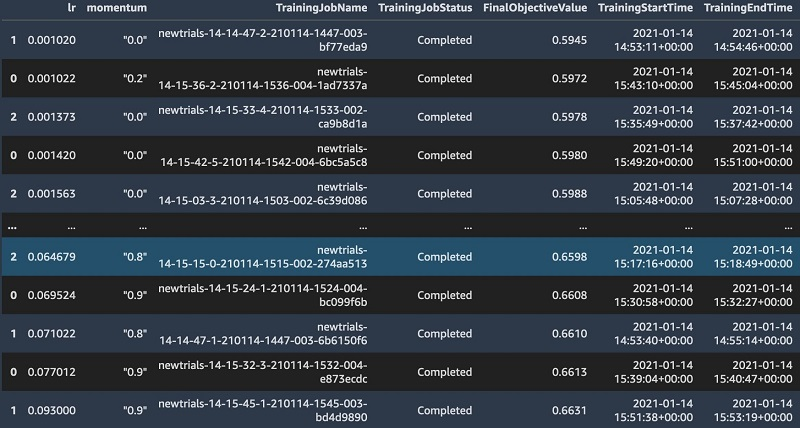The above code will allow you to run HPO jobs in parallel up to the allowed limit of 100 concurrent HPO jobs.

## Parallelizing HPO jobs with warm start

Now suppose you want to run a warm start job, where the result of a prior job is used as input to the next job. Warm start is particularly useful if you have already determined a set of hyperparameters that produce a good model but now have new data. Another use case for warm start is when a single HPO job can take a long time, particularly for deep learning workloads. In that case, you may want to use the outputs of the prior job to launch the next one. For our use case, that could occur when you get a batch of new monthly or quarterly default data. For more information about SageMaker HPO with warm start, see Run a Warm Start Hyperparameter Tuning Job.

The crucial difference between warm and cold start is the naturally sequential nature of warm start. Again, suppose we want to launch 10,000 jobs with warm start. This time, we only launch a single HPO job with the maximally allowed `max_jobs` parameter, wait for its completion, and launch the next job with this job as parent. We repeat the process until the total desired number of jobs is reached. We can achieve this with the following code:

``````def large_scale_hpo_warmstart(job_name_prefix, inputs, max_total_trials,  max_parallel_per_job, max_trials_per_hpo_job=250):
"""Kicks off sequential HPO jobs with warmstart.
job_name_prefix: user defined prefix to name your HPO jobs. HPO will add a timestamp
inputs: locations of train and test datasets in S3 provided as a dict
max_total_trials: total number of trials you want to run
max_trials_per_hpo_job: Fixed at 250 unless you want fewer.
max_parallel_per_job: max trails to run in parallel per HPO job"""

if max_trials_per_hpo_job >250:
raise ValueError('Please select a value less than or equal to 250 for max_trials_per_hpo_job')

base_hpo_job_name = job_name_prefix
timestamp_suffix = strftime("%d-%H-%M-%S", gmtime())
tuning_job_name = lambda i : f"{base_hpo_job_name}-{timestamp_suffix}-{i}"
current_jobs_completed = 0
job_names_list = []
while current_jobs_completed < max_total_trials:
jobs_to_launch = min(max_total_trials - current_jobs_completed, max_trials_per_hpo_job)

hpo_job_config = dict(
estimator=estimator,
objective_metric_name=objective_metric_name,
metric_definitions=metric_definitions,
hyperparameter_ranges=hyperparameter_ranges,
max_jobs=jobs_to_launch,
strategy="Bayesian",
objective_type=objective_type,
max_parallel_jobs=max_parallel_per_job,
)

if current_jobs_completed > 0:
parent_tuning_job_name = tuning_job_name(current_jobs_completed)
warm_start_config = WarmStartConfig(
WarmStartTypes.IDENTICAL_DATA_AND_ALGORITHM,
parents={parent_tuning_job_name}
)
hpo_job_config.update(dict(
base_tuning_job_name=parent_tuning_job_name,
warm_start_config=warm_start_config
))

tuner = HyperparameterTuner(**hpo_job_config)
tuner.fit(
inputs,
job_name=tuning_job_name(current_jobs_completed + jobs_to_launch),
logs=True,
)
tuner.wait()
job_names_list.append(tuner.latest_tuning_job.name)
current_jobs_completed += jobs_to_launch
return job_names_list``````

After the jobs run, again use the `get_best_job` function to aggregate the findings.

## Using other HPO tools with SageMaker

SageMaker offers the flexibility to use other HPO tools such as the ones discussed earlier to run your HPO jobs by removing the undifferentiated heavy lifting of managing the underlying infrastructure. For example, a popular open-source HPO tool is Ray Tune , which is a Python library for large-scale HPO that supports most of the popular frameworks such as XGBoost, MXNet, PyTorch, and TensorFlow. Ray integrates with popular search algorithms such as Bayesian, HyperOpt, and SigOpt, combined with state-of-the-art schedulers such as Hyperband or ASHA.

To use Ray with PyTorch, you first need to include ray[tune] and tabulate to your requirements.txt file in your code folder containing your training script. Provide the code folder into the SageMaker PyTorch estimator as follows:

``````from sagemaker.pytorch import PyTorch

estimator = PyTorch(entry_point="train_ray_cpu.py", #put requirements.txt file to install ray
role=role,
source_dir='./code',
framework_version='1.6.0',
py_version='py3',
output_path = f's3://{bucket}/{prefix}/output',
instance_count=1,
instance_type='ml.m5.xlarge',
sagemaker_session=sagemaker_session,
hyperparameters={
'epochs': 7,
'backend': 'gloo' # gloo for CPU and nccl for GPU
},
disable_profiler=True)

inputs ={'training': train_s3,
'testing':test_s3}

estimator.fit(inputs, wait=True)``````

Your training script needs to be modified to output your custom metrics to the Ray report generator, as shown in the following code. This allows your training job to communicate with Ray. Here we use the ASHA scheduler to implement early stopping:

``````# additional imports
from ray.tune import CLIReporter
from ray.tune.schedulers import ASHAScheduler

# modify test function to output to ray tune report.
# same as test function above with 1 line of code added to enable communication
# with tune.
tune.report(loss=test_loss, accuracy=correct / (len(target)*i), f1score=f1score, roc=roc)``````

You also need to checkpoint your model at regular intervals:

``````for epoch in range(1, args.epochs + 1):
model.train()
for batch_idx, (data, target) in enumerate(train_loader, 1):
data, target = data.to(device), target.to(device)
output = model(data)
loss = F.nll_loss(output, target.squeeze())
loss.backward()

optimizer.step()
if batch_idx % args.log_interval == 0:
logger.info("Train Epoch: {} [{}/{} ({:.0f}%)] Loss: {:.6f}".format(
100. * batch_idx / len(train_loader), loss.item()))
# have your test function publish metrics to tune.report
with tune.checkpoint_dir(epoch) as checkpoint_dir: # modified to store checkpoint after every epoch.
path = os.path.join(checkpoint_dir, "checkpoint")
torch.save((model.state_dict(), optimizer.state_dict()), path)
``````

Finally, you need to wrap the training script in a custom main function that sets up the hyperparameters such as the learning rate, the size of the first and second hidden layers, and any additional hyperparameters you want to iterate over. You also need to use a scheduler, such as the ASHA scheduler we use here, for single- and multi-node GPU training. We use the default tuning algorithm Variant Generation, which supports both random (shown in the following code) and grid search, depending on the config parameter used.

``````def main(args):
config = {
"l1": tune.sample_from(lambda _: 2**np.random.randint(2, 9)),
"l2": tune.sample_from(lambda _: 2**np.random.randint(2, 9)),
"lr": tune.loguniform(1e-4, 1e-1)
}
scheduler = ASHAScheduler(
metric="loss",
mode="min",
max_t=args.epochs,
grace_period=1,
reduction_factor=2)
reporter = CLIReporter(
metric_columns=["loss","training_iteration", "roc"])

# run the HPO job by calling train
print("Starting training ....")
result = tune.run(
partial(train_ray, args=args),
resources_per_trial={"cpu": args.num_cpus, "gpu": args.num_gpus},
config=config,
num_samples=args.num_samples,
scheduler=scheduler,
progress_reporter=reporter)``````

The output of the job looks like the following screenshot.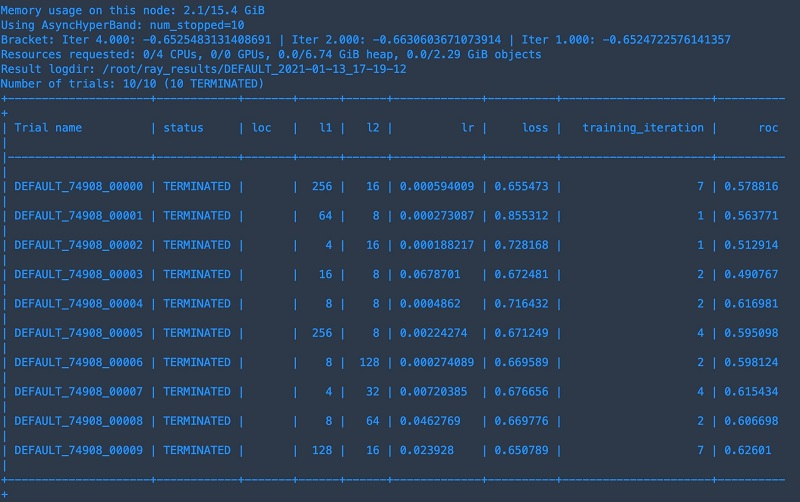Ray Tune automatically ends poorly performing jobs while letting the better-performing jobs run longer, optimizing your total HPO times. In this case, the best-performing job ran all full 7 epochs, whereas other hyperparameter choices were stopped early. To learn more about how early stopping works with SageMaker HPO see here.

## Queuing HPO jobs with Amazon SQS

When multiple data scientists create HPO jobs in the same account at the same time, the limit of 100 concurrent HPO jobs per account might be reached. In this case, we can use Amazon SQS to create an HPO job queue. Each HPO job request is represented as a message and submitted to an SQS queue. Each message contains hyperparameters and tunable hyperparameter ranges in the message body. A Lambda function is also created. The function first checks the number of HPO jobs in progress. If the 100 concurrent HPO jobs limit isn’t reached, it retrieves messages from the SQS queue and creates HPO jobs as stipulated in the message. The function is triggered by Amazon EventBridge events at a regular interval (for example, every 10 minutes). The simple architecture is shown as follows.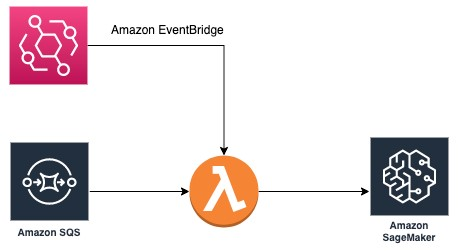To build this architecture, we first create an SQS queue and note the URL. In the Lambda function, we use the following code to return the number of HPO jobs in progress:

``````sm_client = boto3.client('sagemaker')

def check_hpo_jobs():
response = sm_client.list_hyper_parameter_tuning_jobs(
MaxResults=HPO_LIMIT,
StatusEquals='InProgress')
return len(list(response["HyperParameterTuningJobSummaries"]))``````

If the number of HPO jobs in progress is greater than or equal to the limit of 100 concurrent HPO jobs (for current limits, see Amazon SageMaker endpoints and quotas), the Lambda function returns 200 status and exits. If the limit isn’t reached, the function calculates the number of HPO jobs available for creation and retrieves the same number of messages from the SQS queue. Then the Lambda function extracts hyperparameter ranges and other data fields for creating HPO jobs. If the HPO job is created successfully, the corresponding message is deleted from the SQS queue. See the following code:

``````def lambda_handler(event, context):

# first: check HPO jobs in progress
hpo_in_progress = check_hpo_jobs()

if hpo_in_progress >= HPO_LIMIT:
return {
'statusCode': 200,
'body': json.dumps('HPO concurrent jobs limit reached')
}
else:
hpo_capacity = HPO_LIMIT - hpo_in_progress
container = image_uris.retrieve("xgboost", region, "0.90-2")
train_input = TrainingInput(f"s3://{bucket}/{key_prefix}/train/train.csv", content_type="text/csv")
validation_input = TrainingInput(f"s3://{bucket}/{key_prefix}/validation/validation.csv", content_type="text/csv")

while hpo_capacity > 0:
if 'Messages' in sqs_response.keys():
msgs = sqs_response['Messages']
for msg in msgs:
try:
create_hpo(container,train_input,validation_input,hp_in_msg)
response = sqs.delete_message(QueueUrl=queue_url,ReceiptHandle=msg['ReceiptHandle'])
hpo_capacity = hpo_capacity-1
if hpo_capacity == 0:
break
except :
return ("error occurred for message {}".format(msg['Body']))
else:
return {'statusCode': 200, 'body': json.dumps('Queue is empty')}

return {'statusCode': 200,  'body': json.dumps('Lambda completes')}
``````

After your Lambda function is created, you can add triggers with the following steps:

1. On the Lambda console, choose your function.
2. On the Configuration page, choose Add trigger.
3. Select EventBridge (CloudWatch Events).
4. Choose Create a new rule.
5. Enter a name for your rule.
6. Select Schedule expression.
7. Set the rate to 10 minutes.

This rule triggers our Lambda function every 10 minutes.

When this is complete, you can test it out by sending messages to the SQS queue with your HPO job configuration in the message body. The code and notebook for this architecture is on our GitHub repo. See the following code:

``````response = sqs.send_message(
QueueUrl=queue_url,
DelaySeconds=1,
MessageBody=(
'{"hyperparameter_ranges":{
"<hyperparamter1>":<range>,
"hyperparamter2":<range>} }'
)
)``````

## Conclusions

ML engineers often need to search through a large hyperparameter space to find the best-performing model for their use case. For complex deep learning models, where individual training jobs can be quite time consuming, this can be a cumbersome process that can often take weeks or months of developer time.

In this post, we discussed how you can maximize the number of tuning jobs you can launch in parallel with SageMaker, which reduces the total time it takes to run HPO with custom user-specified objective metrics. We first discussed a Jupyter notebook based approach that can be used by individual data scientists for research and experimentation workflows. We also demonstrated how to use an SQS queue to allow teams of data scientists to submit more jobs. SageMaker is a highly flexible platform, allowing you to bring your own HPO tool, which we illustrated using the popular open-source tool Ray Tune.

To learn more about bringing other algorithms such as genetic algorithms to SageMaker HPO, see Bring your own hyperparameter optimization algorithm on Amazon SageMaker.

## References

 Hyper-Parameter Optimization: A Review of Algorithms and Applications, Yu, T. and Zhu, H., https://arxiv.org/pdf/2003.05689.pdf.

 Tune: A research platform for distributed model selection and training, https://arxiv.org/abs/1807.05118.Iaroslav Shcherbatyi is a Machine Learning Engineer at Amazon Web Services. His work is centered around improvements to the Amazon SageMaker platform and helping customers best use its features. In his spare time, he likes to catch up on recent research in ML and do outdoor sports such as ice skating or hiking.Enrico Sartorello is a Sr. Software Development Engineer at Amazon Web Services. He helps customers adopt machine learning solutions that fit their needs by developing new functionalities for Amazon SageMaker. In his spare time, he passionately follows his soccer team and likes to improve his cooking skills.Tushar Saxena is a Principal Product Manager at Amazon, with the mission to grow AWS’ file storage business. Prior to Amazon, he led telecom infrastructure business units at two companies, and played a central role in launching Verizon’s fiber broadband service. He started his career as a researcher at GE R&D and BBN, working in computer vision, Internet networks, and video streaming.Stefan Natu is a Sr. Machine Learning Specialist at Amazon Web Services. He is focused on helping financial services customers build end-to-end machine learning solutions on AWS. In his spare time, he enjoys reading machine learning blogs, playing the guitar, and exploring the food scene in New York City.Qingwei Li is a Machine Learning Specialist at Amazon Web Services. He received his PhD in Operations Research after he broke his advisor’s research grant account and failed to deliver the Nobel Prize he promised. Currently, he helps customers in the financial service and insurance industry build machine learning solutions on AWS. In his spare time, he likes reading and teaching.